Examples

Chapter 10 Class 11 Straight Lines
Serial order wise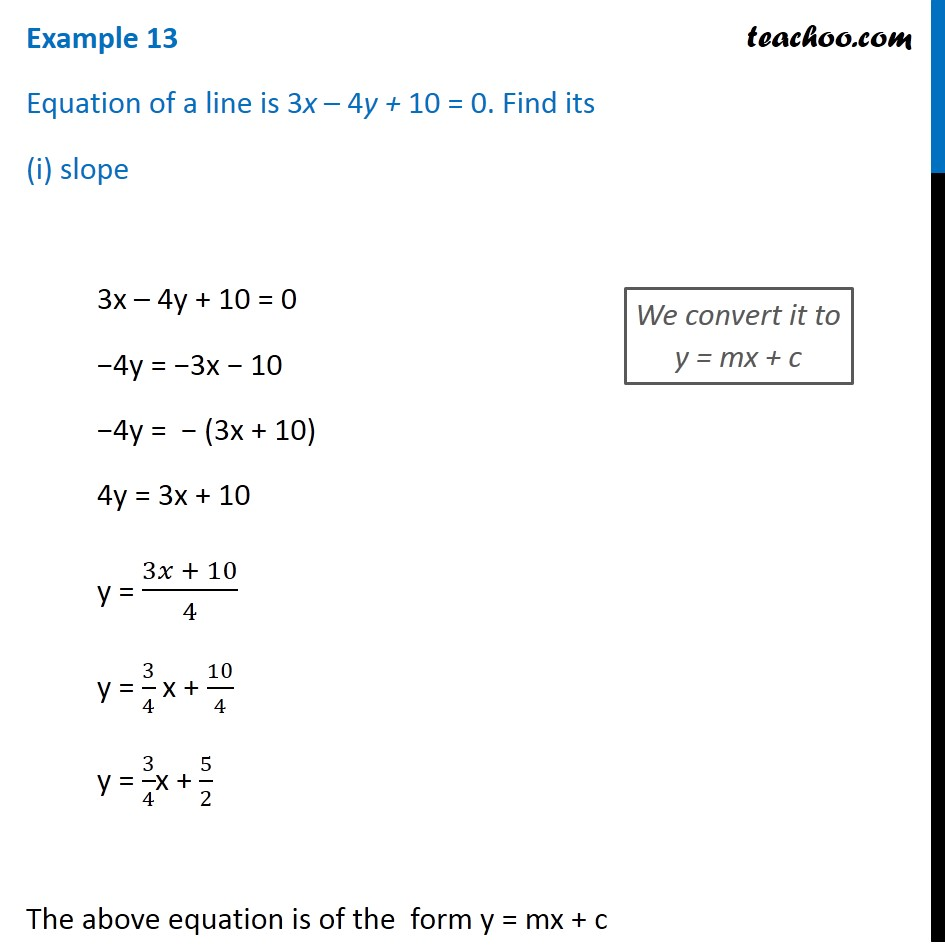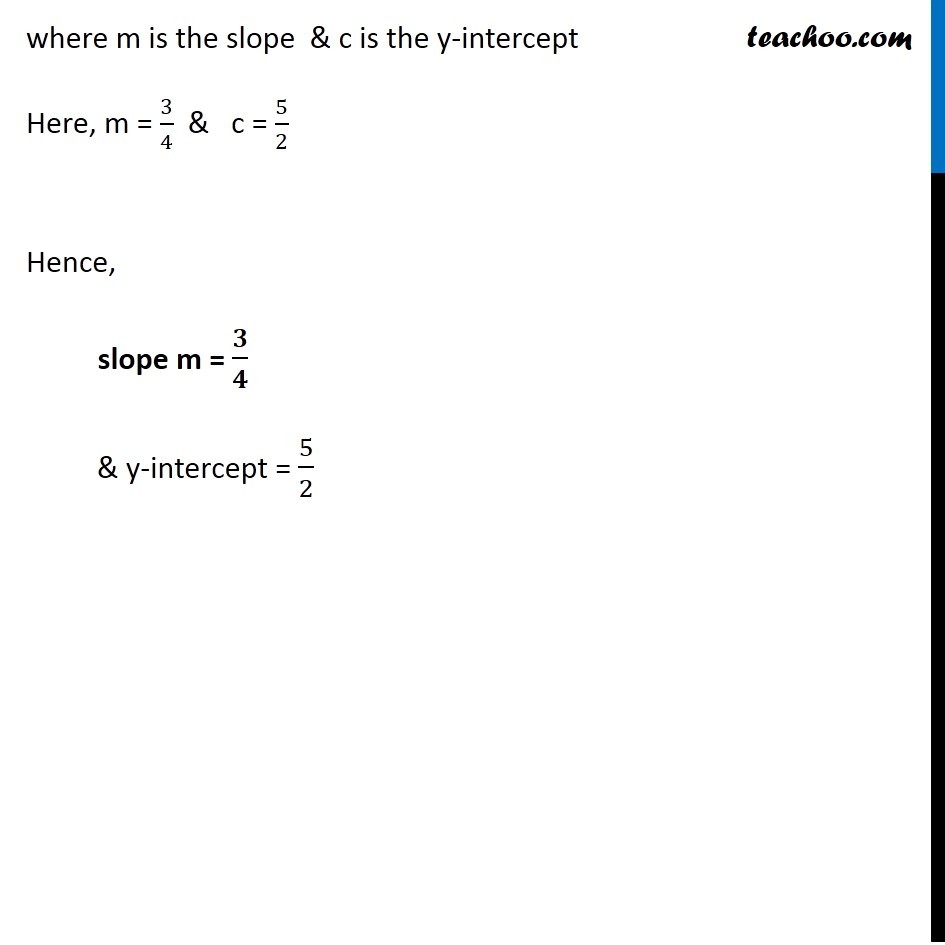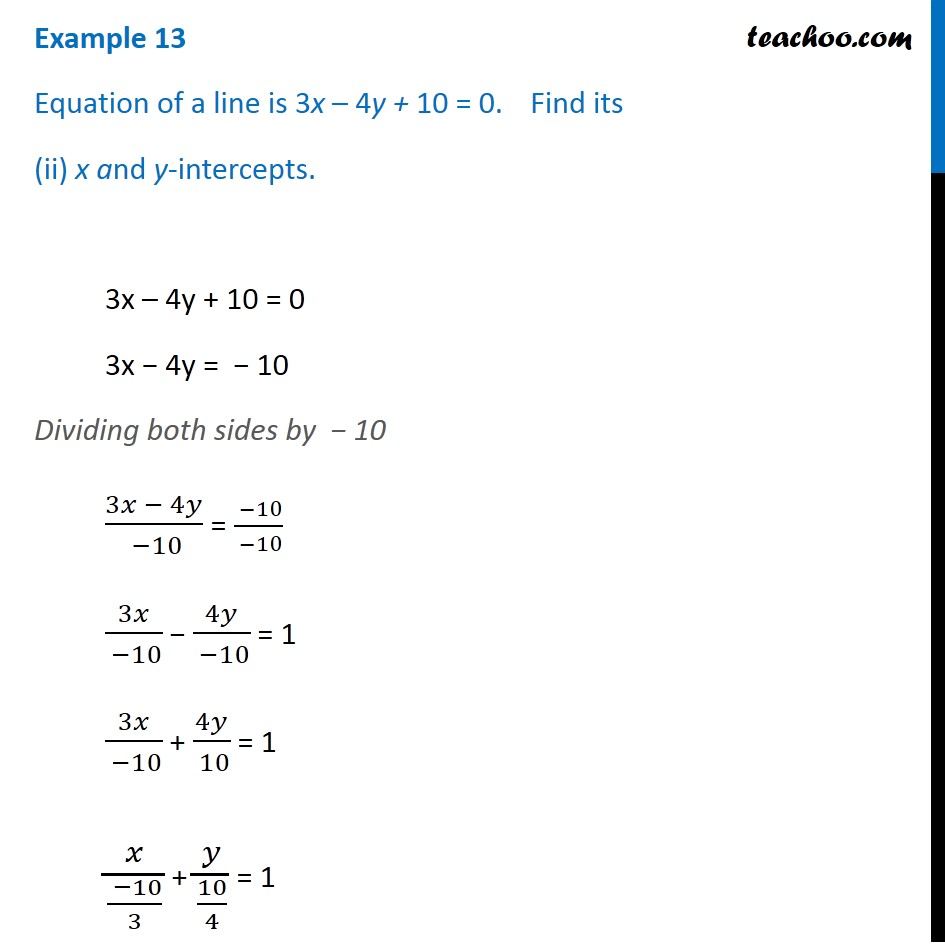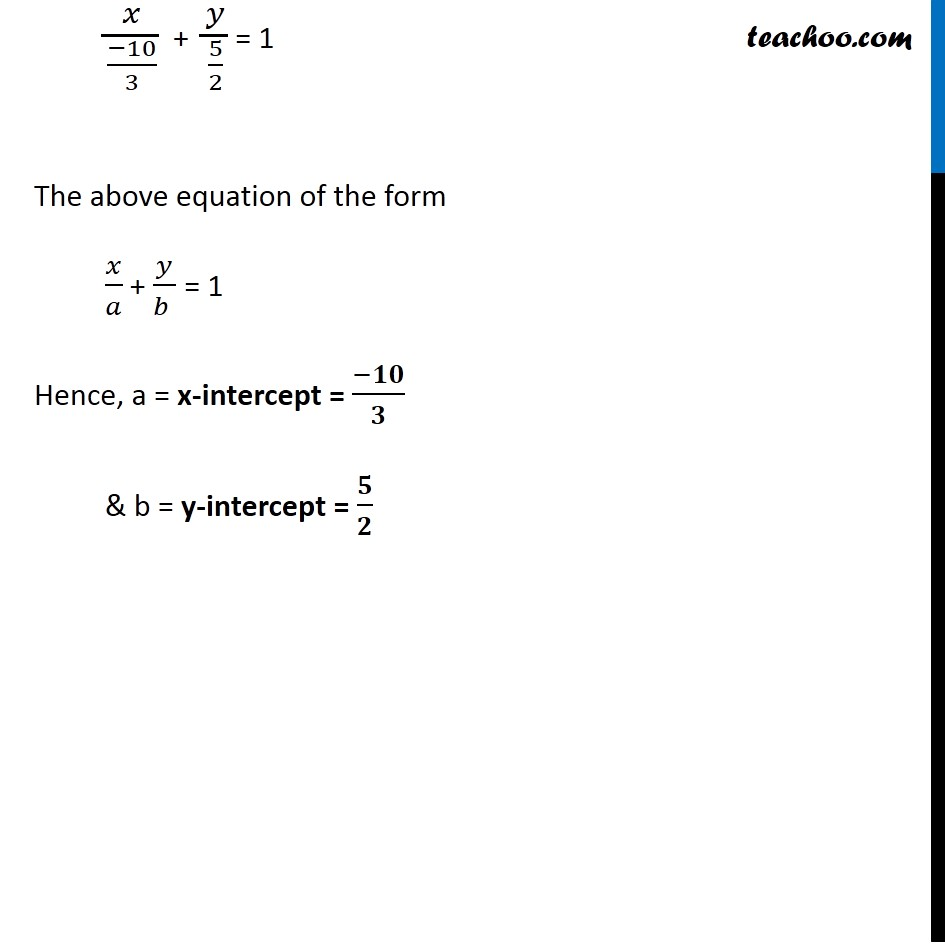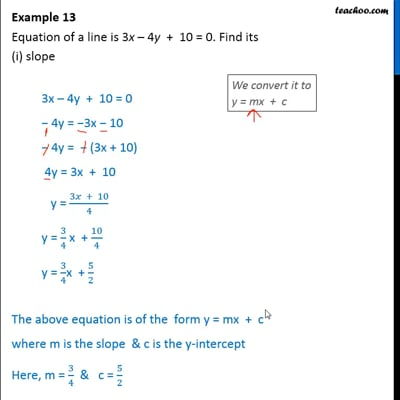This video is only available for Teachoo black users

Solve all your doubts with Teachoo Black (new monthly pack available now!)

### Transcript

Example 13 Equation of a line is 3x – 4y + 10 = 0. Find its (i) slope 3x – 4y + 10 = 0 −4y = −3x − 10 −4y = − (3x + 10) 4y = 3x + 10 y = (3𝑥 + 10)/4 y = 3/4 x + 10/4 y = 3/4x + 5/2 The above equation is of the form y = mx + c We convert it to y = mx + c where m is the slope & c is the y-intercept Here, m = 3/4 & c = 5/2 Hence, slope m = 𝟑/𝟒 & y-intercept = 5/2 Example 13 Equation of a line is 3x – 4y + 10 = 0. Find its (ii) x and y-intercepts. 3x – 4y + 10 = 0 3x − 4y = − 10 Dividing both sides by − 10 (3𝑥 − 4𝑦)/( −10) = ( −10)/( −10) 3𝑥/( −10) − 4𝑦/( −10) = 1 3𝑥/( −10) + 4𝑦/( 10) = 1 𝑥/(( −10)/3) + 𝑦/(10/4) = 1 𝑥/((−10)/3) + 𝑦/(5/2) = 1 The above equation of the form 𝑥/𝑎 + 𝑦/(𝑏 ) = 1 Hence, a = x-intercept = (−𝟏𝟎)/𝟑 & b = y-intercept = 𝟓/𝟐